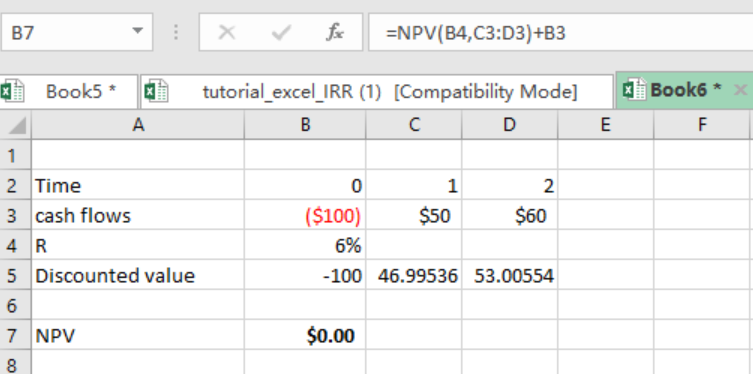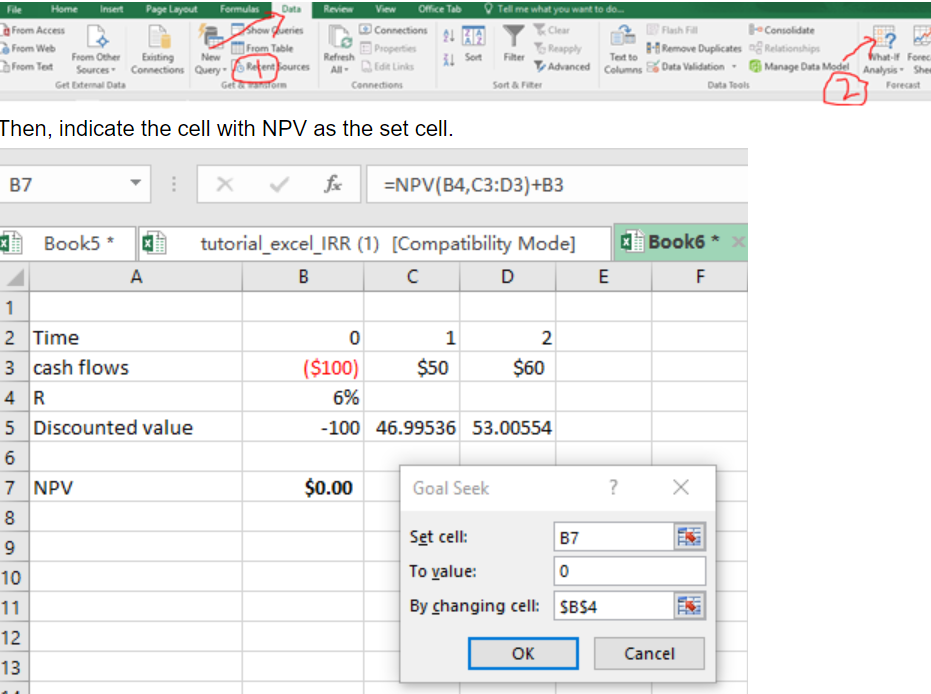Get instant live expert help with Excel or Google Sheets“My Excelchat expert helped me in less than 20 minutes, saving me what would have been 5 hours of work!”

#### Post your problem and you’ll get expert help in seconds.

Your message must be at least 40 characters
Our professional experts are available now. Your privacy is guaranteed.

# Learn how to Calculate Your Money Weighted Rate of Return in Excel

Before we look at how to calculate your money weighted rate of return in excel, it is important to first understand what it means.

## What is money weighted rate of return?

• This is simply a measure of the performance of an asset or portfolio of assets. To calculate the weighted money return you need to find the rate that will set the value of the present values of all cash flows and terminal values equal to the value of initial investment. In other words, the money-weighted rate of return, (MWRR) is equivalent to the internal rate of return (IRR).
• In other words, MWRR is the discount rate at which the net present value or NPV=0. You can also say that it is the discount rate at which present value of all cash inflows equals present value of all cash outflows.
• To understand how we can implement this in real calculations, let us consider the example below;

## ExampleFigure 1

• In the above example, we have found the MWRR to be 6%.
• At 6%, our NPV is zero. In this analysis, our cash flow is \$100, which is the initial investment.
• The cash inflows are \$50 and \$60. Row 5 provides the discounted values, which can help us get the NPV.
• But we can still get the NPV using the NPV function as shown in cell B7.
• To get the interest that can make cash outflow and inflow equal so that we have NPV=0, we might need to use trial and error method, which is tiresome.
• In the example, we have used the GOAL SEEK Excel built-in function to get a percentage that can make our NPV 0.
• To get the goal seek, we proceed as follows;
• Head to the Data in the menu bar.
• Click on What-If Analysis
• Click “Goal Seek”Figure 2

Then, indicate the cell with NPV as the set cell.

Put the set cell value as zero, by changing the cell with the rate. In our case, our set cell is B7, we change it to 0 and we are changing cell B4.

Most of the time, the problem you will need to solve will be more complex than a simple application of a formula or function. If you want to save hours of research and frustration, try our live Excelchat service! Our Excel Experts are available 24/7 to answer any Excel question you may have. We guarantee a connection within 30 seconds and a customized solution within 20 minutes.

Solution examplesI have a figure which is the sum of 9 months of payments. Each payment decreases by the same amount (X) so the first payment is Y, second payment is Y-X, third is Y-(Xx2), forth is Y-(Xx3) and so on. I am trying to calculate what each of the 9 payments should be.
Solved by A. W. in 60 mins